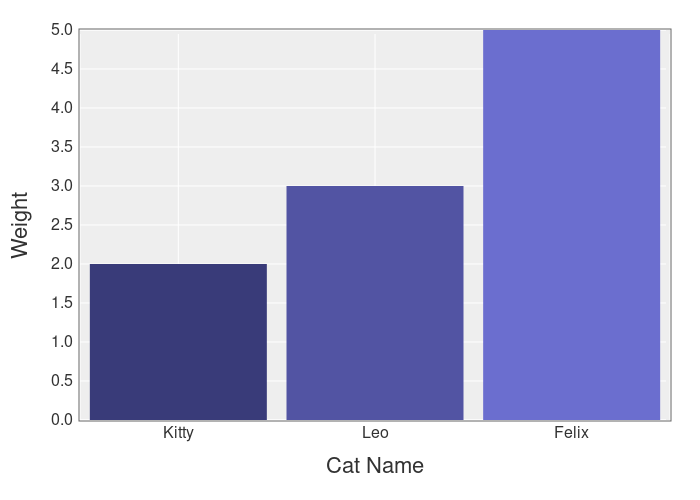# What is A Matrix & How to Use It in Ruby?

A matrix is a 2D (2-dimensional) array that can be used to store & work with spreadsheet-like data.

They can be used for:

• Representing a board in a table game (chess, checkers, etc.)
• Statistics & data analysis
• Generating plots & graphs

Because this is a powerful data structure it’s helpful to learn how to use it.

## How to Create a Matrix in Ruby

You can create a matrix with arrays.

Like this:

```matrix = [
[1,2,3],
[4,5,6],
[7,8,9]
]
```

This produces a 3×3 matrix & it’s your best choice if you want to store 2-dimensional data as a board or set of positions.

But if you would like to combine matrices via addition, subtraction & multiplication…

Then you can use the `Matrix` class.

Here’s how to use it:

```require 'matrix'

a = Matrix[[1,2,3], [4,5,6], [7,8,9]]
b = Matrix[[1,2,3], [4,5,6], [7,8,9]]
```

```a + b
# Matrix[[2, 4, 6], [8, 10, 12], [14, 16, 18]]
```

You can access individual values like this:

```a[0, 1]
```

This is different from the array version, which would use this syntax:

```matrix
```

Both array & matrix can be transposed, that means that the rows become columns & the columns become rows.

Example:

```matrix.transpose

# [[1, 4, 7], [2, 5, 8], [3, 6, 9]]
```

Also remember:

Matrix objects are immutable, so you can’t change the values without creating a new matrix. This `Matrix` is mostly for mathematical operations, if you want data analysis & statistics you’ll need something more powerful.

Something like…

## The Daru Gem

Daru is a gem that allows you to work with matrices, get statistics from them & print them as a nicely formatted table. Daru also integrates with Ruby plotting gems so you can generate plots & graphs from your data.

Here’s an example:

```require 'daru'

df = Daru::DataFrame.new(
{
"A" => [1,2,3],
"B" => [4,5,6],
"C" => [7,8,9]
},
index: ["A", "B", "C"]
)
```

This prints the following table:

```    A   B   C
A   1   4   7
B   2   5   8
C   3   6   9
```

You can access a specific column like this:

```df['A']
```

Or by numerical index:

```df
```

And you can get statistics like this:

```df['B'].describe

#         statistics
# count            3
# mean           5.0
# std            1.0
# min              4
# max              6
```

The best part?

You can load data directly from CSV files, ActiveRecord & even Excel files.

Example:

```df = Daru::DataFrame.from_csv('healthy_food.csv')
```

And you can filter the data with a `where` expression.

For example…

If we have a “carbs” column, we can find all the rows in our matrix that have a value of less than 25.

Like this:

```df.where(df['carbs'].lt(25))
```

You can also `sort`, `group_by` & `aggregate` your data frames.

Example:

```df = Daru::DataFrame.new(
{  str: %w(a b c d a),
num: [52,12,7,17,1] }
)

df.group_by(:str).aggregate(num: :sum)

#    num
# a   53
# b   12
# c    7
# d   17
```

## Plotting With Daru

Daru allows you to create visual representations of your data & export them as HTML files.

Here’s an example:

```df = Daru::DataFrame.new(
{'Cat Names' => %w(Kitty Leo Felix),
'Weight'   => [2,3,5]}
)

df.plot(type: :bar, x: 'Cat Names', y: 'Weight') do |plot, _|
plot.x_label 'Cat Name'
plot.y_label 'Weight'

plot.yrange [0, 5]
end
.export_html
```

This produces this chart:You’ll find this chart as an HTML file in the same folder as your code.

If you want to use Daru in your Rails application you’ll need to add the `daru-view` gem to the mix or use a different gem like chartkick.

## Summary

You have learned about matrices in Ruby so you can work with 2-dimensional data!